# Help understanding transistor as a switch

#### Robartes

Joined Oct 1, 2014
57
I'm still struggling with understanding basic transistor circuits -- I'm working on it, and I know from experience that one day it will 'click' and everything will fall into place -- hopefully before I grow too old and forget what transistors are. However, I'm not there yet and yesterday this bit me when I tested a naive transistor switch circuit and it did not work. I think I have figured out why it does not work and how I can fix it. I'm posting this here to see if my understanding is correct, so please critique where necessary.

The circuit is a very basic transistor switch where a 5V logic signal needs to switch a 12V LED strip segment (three RBG LEDS in series). My attempt, the one that does not work, is naive simplicity itself: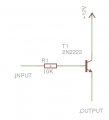Input is a 5V signal, output goes to the anode of the LEDs (they drop around 10V and draw 70mA doing so). I was stumped when I powered this up and nothing happened. After a bit of head scratching, I broke out the multimeter and started measuring some voltages on the transistor:

Vb = 4.2V
Ve = 3.6 V
Vc = 12V

Doh! Of course this does not work. The emitter sits one diode drop below the base, which in this case means 3.6V, far from the 12V needed for the LEDs. In fact I'm lucky I did not smoke the transistor, as it was dissipating over half a watt (current to the LEDs is 70mA).

This is what I mean by struggling with understanding transistor circuits - anyone with a better (or any) understanding of transistors would have seen immediately that this will not work without having to actually try it.

Anyway, to get this to work I see two solutions.

- Option 1: take the output from the collector of the transistor and make it a sort of common emitter amplifier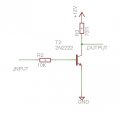This has two disadvantages. One, I would have to invert the logic of my software (this is part of a 4x4 matrix controlled by a microcontroller). That is not too much of a problem. However, the second problem might be a bit more serious. This is where I'm treading on even shakier ground, so please follow along with my calculations and correct where wrong.

Assuming I still want 11V on Vout (I tested the LEDs and they still work at that voltage) when the transistor is off, the resistor would need to be 1V / 0.07A or 70 ohm, and will dissipate 70mW. So far so good. Now, when the transistor is on, things get a bit hotter.

When the transistor is on, Vb is 4.2V and Ve is 3.6V (see above). Assuming the worst case scenario where the transistor is fully saturated (and this is the part I understand least - will it be saturated? ), Vc will also be 3.6V (actually a bit more, but we're assuming worst case). Rc will thus drop 12V - 3.6V = 8.4V . Dropping 8.4V across a 70 ohm resistor results in a current of 120mA and will dissipate just over 1W of power. So, in order to use this solution, I would need power resistors - not what I expected.

- Option 2: do what I should have done from the start and use FETs instead of bipolar junction transistors. At least I'm assuming that using a logic level FET would actually make this work - is that correct?

#### camjerlams

Joined Dec 23, 2012
56
I'm still struggling with understanding basic transistor circuits -- I'm working on it, and I know from experience that one day it will 'click' and everything will fall into place -- hopefully before I grow too old and forget what transistors are. However, I'm not there yet and yesterday this bit me when I tested a naive transistor switch circuit and it did not work. I think I have figured out why it does not work and how I can fix it. I'm posting this here to see if my understanding is correct, so please critique where necessary.
I'm learning transistors too, so just lurking atm.
I would have thought this circuit would be fine, I would sink the current though, LEDs on the 12v side but I cant see how that would stop things from working.
It would be nice if someone could give an explanation of how to use the datasheet V(CB), V(CE) and V(EB) in selecting a transistor, is this the maximum voltage allowed between these pins?

#### blocco a spirale

Joined Jun 18, 2008
1,546
Why do you think you need a 70 Ohm resistor on the collector?

#### Robartes

Joined Oct 1, 2014
57
Why do you think you need a 70 Ohm resistor on the collector?
The LEDs draw 70mA and I wanted a 1V drop across the resistor to leave 11V on the output. The rest is Ohm's law (or my misapplication of it).

#### blocco a spirale

Joined Jun 18, 2008
1,546
You show the 70 Ohm resistor connected between 12V and the resistor collector, is this your intent?

If so, you have created a 2W heater every time the transistor switches on, what has this to do with the LEDs?

Let's try and get things back in order, forget everything you have already done: What is the forward voltage and current of each of your LEDs which will be driven from this transistor?

#### djsfantasi

Joined Apr 11, 2010
9,128
I've always switched my LEDs with an NPN transistor, with the LEDs in series between the collector and the power source, like the picture following.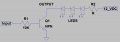The problem I see with these circuits depends on the characteristics of the LEDs you are using. Taking a typical value for an RGB LED, Vf can be 3.5-3.6 volts. Calculating the resistor value needed for the voltage to be dropped to keep current at 70 mA, one uses Ohms law:
$$\frac{(12-(3*V_f+0.7))}{0.07}$$
If $$V_f$$ is 3.6 volts, the numerator evaluates to 0,5 volts. This is too low to reliably use a current limiting resistor. Series groups of two LEDs will work better, use a transistor per LED or raise the power supply.

#### Robartes

Joined Oct 1, 2014
57
If so, you have created a 2W heater every time the transistor switches on, what has this to do with the LEDs?

Let's try and get things back in order, forget everything you have already done: What is the forward voltage and current of each of your LEDs which will be driven from this transistor?
Good question, difficult answer. The 'LED' is actually a portion of an RGB LED strip. It contains 6 5050 SMD RGB LEDs with common anode, and has connections for +12V, R, G and B. I don't know whether they are wired in series or parallel inside the strip (though I assume serial). When I power it with 12V on the anode and all three cathodes connected to ground, it draws 76,9mA (just measured). As to individual voltage drop per LED, I can't really measure that at the moment without hacking open the strip. This might clarify things a bit: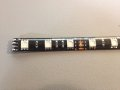You show the 70 Ohm resistor connected between 12V and the resistor collector, is this your intent?
Yes, though quite possibly that's a mistake. Should the resistor be elsewhere, or should it even be there?

#### Robartes

Joined Oct 1, 2014
57
I've always switched my LEDs with an NPN transistor, with the LEDs in series between the collector and the power source, like the picture following.
I have that type of circuit in the ground lines of each of the LED strip segments. I have 16 of these strip pieces. The R, G and B lines of all 16 LED strip segments are connected together and then switched through a Darlington NPN transistor each, which are driven by a PWM signal for the color. I want to also switch each segment on or off individually however, that's why I need the switch in the cathodes anodes as well.

Edit: corrected 'cathodes' to 'anodes'

Last edited:

#### Robartes

Joined Oct 1, 2014
57
I have that type of circuit in the ground lines of each of the LED strip segments. I have 16 of these strip pieces. The R, G and B lines of all 16 LED strip segments are connected together and then switched through a Darlington NPN transistor each, which are driven by a PWM signal for the color. I want to also switch each segment on or off individually however, that's why I need the switch in the cathodes as well.
Here's a quick sketch of what I mean: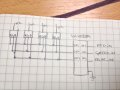Each little square is a LED strip segment, the chip is a ULN2804 Darlington array. The full monty is with 16 segments, but the idea is the same. This works if I connect the anodes to +12V directly, but I want to switch them with a transistor.

Edit: and to further clarify, each of the +12V will get its own transistor, so I can switch them individually.

Last edited:

#### blocco a spirale

Joined Jun 18, 2008
1,546
It appears that the LED strip includes resistors and is designed to work directly from 12V. In which case you can simply connect the module in place of your 70 Ohm resistor (you don't need the 70R resistor). If you want individual control of RGB you will, obviously, need one transistor per LED.

#### Robartes

Joined Oct 1, 2014
57
I also recommend the common emitter configuration as in djsfantasi's example above but consider the value of your base resistor.

http://www.electronics-tutorials.ws/transistor/tran_4.html
Thanks for that link - it helped. Looking through that page, I think I might have figured it out now. The problem I had/have with the common emitter configuration is that I cannot just insert the LED strips in the collector line of an NPN transistor, as I also need to put the three individual PWM signals somewhere. The page you linked however shows a PNP common emitter circuit which seems to be what I am looking for. Instead of NPN I think I can use PNP transistors to source the current to the +12V of the LED segment. The current is then sunk into the Darlington array at the low end.

#### blocco a spirale

Joined Jun 18, 2008
1,546
Thanks for that link - it helped. Looking through that page, I think I might have figured it out now. The problem I had/have with the common emitter configuration is that I cannot just insert the LED strips in the collector line of an NPN transistor, as I also need to put the three individual PWM signals somewhere. The page you linked however shows a PNP common emitter circuit which seems to be what I am looking for. Instead of NPN I think I can use PNP transistors to source the current to the +12V of the LED segment. The current is then sunk into the Darlington array at the low end.
Why do you think you need a transistor to source current and one to sink current to the LEDs? One transistor is all you to control each LED, if you need one at each end there is probably something wrong/sub-optimal with your design philosophy.

#### Robartes

Joined Oct 1, 2014
57
Why do you think you need a transistor to source current and one to sink current to the LEDs? One transistor is all you to control each LED, if you need one at each end there is probably something wrong/sub-optimal with your design philosophy.
I agree that there is probably something suboptimal in my design philosophy.

The reason I think I need transistors to source and others to sink current is that I need to both switch the +12V lines (to select which of the 16 LED strip segments should light up) and the ground lines (to put a PWM signal on them to select the color of the segment that lights up).

I have wired up the segments with individual connections to each of their +12V and have connected the all R's together, all G's together and all B's together. So I end up with 16 +12V lines and three ground lines to switch, so 16 transistors to source and three to sink current. Refer to my little drawing in post #10 above to make this a bit more clear (or, given my track record in this thread, confuse you even more).

I could have wired up all of the +12V together and switched each of the 16 R, G and B lines individually so I needed only sink transistors (though it would be 48 of them), which might have been a more optimal design.

#### blocco a spirale

Joined Jun 18, 2008
1,546
Ah, I understand now; you are multiplexing the LEDs, this makes sense.

#### WBahn

Joined Mar 31, 2012
29,489
Okay, let's take first things first and do it in stages so that we can more easily see when and where we diverge so that we can get back on track more easily.

It appears that your LED strips are hooked up essentially like the following: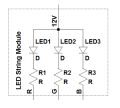And you want to multiplex them so that you can address each LED individually via switches (which will become transistors):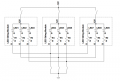You want to control these with 5V logic signals. The bottom switches is not a problem and you can just use NPN transistors with suitable base transistors for that. But the upper switches require a bit of trickery because you need to use PNP signals and you need to get the base voltage up to 12V to turn them off and down to 11.3V to turn them on. You can do this very easily with an extra layer of NPN transistors to control the PNP transistors: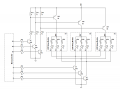Which transistors you use will depend on the total current needs for each one. This will also affect the resistor sizing. If this looks like something that will work for you, we can proceed with those questions.

•#### Robartes

Joined Oct 1, 2014
57
Okay, let's take first things first and do it in stages so that we can more easily see when and where we diverge so that we can get back on track more easily.

It appears that your LED strips are hooked up essentially like the following:

View attachment 77934

And you want to multiplex them so that you can address each LED individually via switches (which will become transistors):

View attachment 77935

You want to control these with 5V logic signals. The bottom switches is not a problem and you can just use NPN transistors with suitable base transistors for that. But the upper switches require a bit of trickery because you need to use PNP signals and you need to get the base voltage up to 12V to turn them off and down to 11.3V to turn them on. You can do this very easily with an extra layer of NPN transistors to control the PNP transistors:

View attachment 77943

Which transistors you use will depend on the total current needs for each one. This will also affect the resistor sizing. If this looks like something that will work for you, we can proceed with those questions.
Yup - that's it.

I had already worked out the problem with the PNP transistors up top and the logic level signal into their bases (5V is about 10 diode drops below the emitter voltage so these transistors are always on) but had not come up with your 'auxiliary NPN' solution yet. Thanks!

Tell you what - it's a bit late in the evening for me to start doing this right now, but let me have a go at working out the transistors & resistor values in your design and I'll report back with my findings here later. Thanks again!

#### WBahn

Joined Mar 31, 2012
29,489
Sounds good. We just got home and I don't plan on being out of bed for another twelve hours or so (can't even go to bed for a few more hours because I'm tending the fireplace until the house warms up enough to keep the furnace from coming on while we sleep). So I'll check back then.

#### Robartes

Joined Oct 1, 2014
57
OK, I found some time between wrapping up gifts and buying crustaceans (don't ask) to come up with these calculations. I am focusing the calculations on the following components in WBahn's schematic in post #15: Q5, R12, R13, Q7 and R16. This way I have one PNP & NPN transistor pair and their supporting resistors - the rest should be the same. Please follow along and correct me where I'm wrong - thanks!

First to determine the values of R12 and R13. To calculate these, I need to know the current through them. To do this, I'm starting with the situation that both Q5 and Q7 are on.

This is my first bit of thin ice. I think the base current of a PNP transistor flows *out* of the base, so in this case from the base of Q5 through R13 and Q7 down to ground. If so (and I hope so because the rest of this post is based on that assumption), the current through R12 is then just the collector current of Q7, IcQ7 and the current through R13 is IcQ7 plus the base current of Q5, IbQ5.

IbQ5 can be assumed to be one tenth of the collector current of Q5 (assume a beta of 10 to ensure full saturation). IcQ5 is the current through a single LED strip segment, which I have measured at 76,9 mA. So:

IbQ5 = IcQ5 / 10 = 7.69mA

For IcQ7 I tread on my second bit of thin ice. I'm going to try this with PN2222 transistors (because I have quite a lot of those lying about), and the datasheet gives me just one data point for Ic vs Vce(sat). It sets Vce(sat) at 1V for 50mA of collector current, so I'm going to arbitrarily set IcQ7 at 50mA. The current through R12 and R13 will then be:

IR12 = IcQ7 = 50mA
IR13 = IcQ7 + IbQ5 = 57,69mA

Next are the voltage drops across R12 and R13. R12 needs to drop one diode drop, as the voltage at the bottom of R12 is also the base voltage of Q5, which needs to be one diode drop below the emitter voltage of Q5, which is the positive rail (+12V). So VR12 is 0.7V. The voltage drop of R13 is then 11.3 - 1 (the bottom of R13 is VcQ7) or 10.3V. So now we can calculate R12 and R13:

R12 = VR12 / IR12 = 0.7 / 0.05 = 14ohm
R13 = VR13 / IR13 = 10.3 / 0.05769 = 178.54 ohm

And power dissipation across them:

P12 = VR12 * IR12 = 0.7*0.05 = 35mW
P13 = VR13 * IR13 = 10.3 *0.05769 = 600mW. That's a lot, so I will probably have to recalculate this with less collector current for Q7, but I'm going to continue with these values for now.

So now there only remains R16, the base resistor of Q7. Again going with a beta of 10, IbQ7 is going to be 5mA. VbQ7 will be one diode drop above VeQ7, which is at ground, so VbQ7 = 0,7V and the voltage drop across R16 will be 4.3V (the other side is a 5V logic signal). So R16 will be:

R16 = VR16 / IR16 = 4.3 / 0.005 = 860 ohm
P16 = 4.3 * 0.005 = 21,5mW

And that's pretty much it for these calculations. For the PNP transistors, I've been having a rummage through my drawers and came up with quite a number of 2N3906 transistors, which have a maximum collector current of 200mA and dissipation of 635mW, so they should do just fine.

Next steps are to recalculate this with a lower IcQ7 to lower dissipation across R13, but I do not have an idea what VcQ7 will become in that case - can I assume that Vce(sat) is linear wrg to Ic, so that for half the Ic, Vce(sat) is half again as well?

#### WBahn

Joined Mar 31, 2012
29,489
OK, I found some time between wrapping up gifts and buying crustaceans (don't ask) to come up with these calculations. I am focusing the calculations on the following components in WBahn's schematic in post #15: Q5, R12, R13, Q7 and R16. This way I have one PNP & NPN transistor pair and their supporting resistors - the rest should be the same. Please follow along and correct me where I'm wrong - thanks!
That's the way to start -- focus in on a minimal set of components at a time. There are a couple of things that affect the calculations -- the first has to do with how you intend to operate the LEDs and the second has to do with the LEDs themselves. Typically, when LEDs or other things are multiplexed this way the intent is to be able to address them separately. If that is the way that they will always be operated, then you never have all three LED strings within a given segment on at the same time. But they are commonly operated in a ganged mode such that the switches one one side are closed one at a time in sequence while the switched on the other side will be turned on in such a way as to activate some subset of the LEDs ranging from none to all. There are two ways of doing this -- walk across the high-side switches or walk across the low-side switches. So we have three options:

Individually: The duty cycle of each LED is 1/(3N) were N is the number of LED segments (what I call a module in my schematic). With N=16, that means that the duty cycle of each LED that is "on" will only be ~2%, which is pretty low. You will have to hit it with a lot of current and walk through the cycle very quickly to make each one appear that it is on steadily at a modest current. But hitting it with a lot of current is not an option because of the internal resistors, so you really need a means of improving the duty cycle.

High-side Sequence: If you walk across the high-side switches one at a time then you are turning on one segment at a time and, for each segment, turning on some subset of the NPN low-side switches. Thus the high-side switches may have to carry the full segment current (~80mA) but the low side switches only have to carry the current for one string at a time, which gives you nice small currents to work with. Notice that this is independent of the number of segments, which allows you to design for one segment and then just copy it for an arbitrary number of segments, which is nice. However, this gives you a duty cycle of 1/N -- which is a factor of three improvement over walking through the LEDs individually -- but with N=16 it is only about 6% which is still pretty low. Since the current is limited internally, each LED will appear about 1/16 as bright as if the segment were on all the time. That probably isn't going to be good enough.

Low-side Sequence: If you walk across the low-side switches one at a time then you are turning on each color in sequence and, for each color, turning on some subset of the segments. Thus the high-side switches only have to carry the current for one LED at a time, but the low-side switches may have to carry the current for up to N LEDs at a time. The consequence is that your design for has to reflect the number of segments, which is unfortunate but not the end of the world. The good news is that your duty cycle for each LED is 33%, which is probably good enough and it is independent of the number of segments.

As with all things engineering, everything involves compromises and which is the "best" compromise depends on what is most important. You will have to decide that, but I'm going to guess that getting as high a duty cycle as possible is going to be high on the list, so I will assume that you will opt for the low-side sequence approach. If I've guessed wrong you should have enough information to make the necessary adjustments to another approach.

The second issue is how the current within a segment is split between the three color strings. The internal resistors were probably sized to so as to achieve equal optical brightness which probably results in different current in each string, so you really need to measure each color separately as one of them may be noticeably higher than the average and the low side switches need to carry N times that current. So let's define some parameters:

N=16 // number of segments
Iseg = 80 mA // current in a single segment when all strings are on (rounding your 76.9 mA up a bit)
Imax = 30 mA // current in the highest current string within a segment (a guess)

So each high-side switch needs to be sized to carry Imax since only one color will be turned on at a time.

Each low-side switch needs to be sized to carry N*Imax since it is possible that all of the segments could be turned on at once. N*Imax is 480 mA so let's just round that to 500 mA.

I'm over the 10,000 character post limit, so I'll split this here and continue in another post.

#### WBahn

Joined Mar 31, 2012
29,489
Continuing on from the past post.

First to determine the values of R12 and R13. To calculate these, I need to know the current through them. To do this, I'm starting with the situation that both Q5 and Q7 are on.

This is my first bit of thin ice. I think the base current of a PNP transistor flows *out* of the base, so in this case from the base of Q5 through R13 and Q7 down to ground. If so (and I hope so because the rest of this post is based on that assumption), the current through R12 is then just the collector current of Q7, IcQ7 and the current through R13 is IcQ7 plus the base current of Q5, IbQ5.

IbQ5 can be assumed to be one tenth of the collector current of Q5 (assume a beta of 10 to ensure full saturation). IcQ5 is the current through a single LED strip segment, which I have measured at 76,9 mA. So:

IbQ5 = IcQ5 / 10 = 7.69mA
This is correct assuming that all three colors could be turned on at once, which would be for a high-side sequence approach.

I don't know which PNP transistor you are planning to use. The usual complement to the 2N2222 NPN is the 2N2907 PNP.

https://www.fairchildsemi.com/datasheets/MM/MMBT2907.pdf

For IcQ7 I tread on my second bit of thin ice. I'm going to try this with PN2222 transistors (because I have quite a lot of those lying about), and the datasheet gives me just one data point for Ic vs Vce(sat). It sets Vce(sat) at 1V for 50mA of collector current, so I'm going to arbitrarily set IcQ7 at 50mA.
There is no reason to operate at a current that high and several reasons not to -- besides, I think you are misreading the data sheet. From:

https://www.fairchildsemi.com/datasheets/PN/PN2222A.pdf

The Vce(sat) has a max of 1V when the BASE current is 50mA. At a collector current of 150 mA Vce(sat) is only 0.3V. You only need a collector current that is 1/10 of the collector current in Q5 plus the current in the pull-up resistor R12, which won't be much at all, so 3 mA should be sufficient giving you a base current of 0.3 mA. You don't want to set this current too high because your microcontroller has to source up to N times the base current for these.

The current through R12 and R13 will then be:

IR12 = IcQ7 = 50mA
IR13 = IcQ7 + IbQ5 = 57,69mA

Next are the voltage drops across R12 and R13. R12 needs to drop one diode drop, as the voltage at the bottom of R12 is also the base voltage of Q5, which needs to be one diode drop below the emitter voltage of Q5, which is the positive rail (+12V). So VR12 is 0.7V. The voltage drop of R13 is then 11.3 - 1 (the bottom of R13 is VcQ7) or 10.3V. So now we can calculate R12 and R13:

R12 = VR12 / IR12 = 0.7 / 0.05 = 14ohm
R13 = VR13 / IR13 = 10.3 / 0.05769 = 178.54 ohm

And power dissipation across them:

P12 = VR12 * IR12 = 0.7*0.05 = 35mW
P13 = VR13 * IR13 = 10.3 *0.05769 = 600mW. That's a lot, so I will probably have to recalculate this with less collector current for Q7, but I'm going to continue with these values for now.
Your basic approach is pretty good and it is very good that you are asking about power and evaluating whether it is too much. But your starting premise is wrong. You want to set the collector current of Q7 based on the base current needs of Q5. The purpose of R12 is just to ensure that Q5 gets turned off when Q7 is off despite the possibility of some leakage current through Q7, which is very low (well under a microamp). So if we allow for 1 uA and want R12 to still pull the base of Q5 to less than, say, 0.1V of the positive rail, then it can be 100 kO. Let's use 10 kO to be ultra conservative since the current through R12 even when Q7 is on is still going to be less than 0.1 mA.

To size R13, we want it to drop (12V-0.7V)-(0.3V)=11V with 3.1 mA flowing in it, so 3.55 kO. Let's use 3.3 kO.

We want the power in all of the components, though at these currents it won't be a problem.

Q5: 0.4V*30mA = 12 mW
R12: (0.7V)²/10kO = 5 mW
R13: 11V*3.1mA = 34 mW
Q7: 0.3V*3.1mA = 1 mW
So now there only remains R16, the base resistor of Q7. Again going with a beta of 10, IbQ7 is going to be 5mA. VbQ7 will be one diode drop above VeQ7, which is at ground, so VbQ7 = 0,7V and the voltage drop across R16 will be 4.3V (the other side is a 5V logic signal). So R16 will be:

R16 = VR16 / IR16 = 4.3 / 0.005 = 860 ohm
P16 = 4.3 * 0.005 = 21,5mW
The reasoning is correct. Adjusting for the base current of Q7 being just 0.3 mA we need R16 to be about 14 kO. We can go down to 10 kO which will give use some more margin while keeping our base current under 0.5mA and the power under 2mW.

And that's pretty much it for these calculations. For the PNP transistors, I've been having a rummage through my drawers and came up with quite a number of 2N3906 transistors, which have a maximum collector current of 200mA and dissipation of 635mW, so they should do just fine.
Those should do fine.

Don't forget that you haven't dealt with the low-side switches, Q1/R19, yet. Since each of those needs to carry 500 mA, which means a base current of 50 mA which is probably going to stress your microcontroller. I would recommend using a Darlington-like configuration and using one NPN switch for each four segments and then driving those, through separate base resistors, with a single NPN from the microcontroller.

Next steps are to recalculate this with a lower IcQ7 to lower dissipation across R13, but I do not have an idea what VcQ7 will become in that case - can I assume that Vce(sat) is linear wrg to Ic, so that for half the Ic, Vce(sat) is half again as well?
It's roughly linear. But using a lower limit of about 200mV is a good idea.